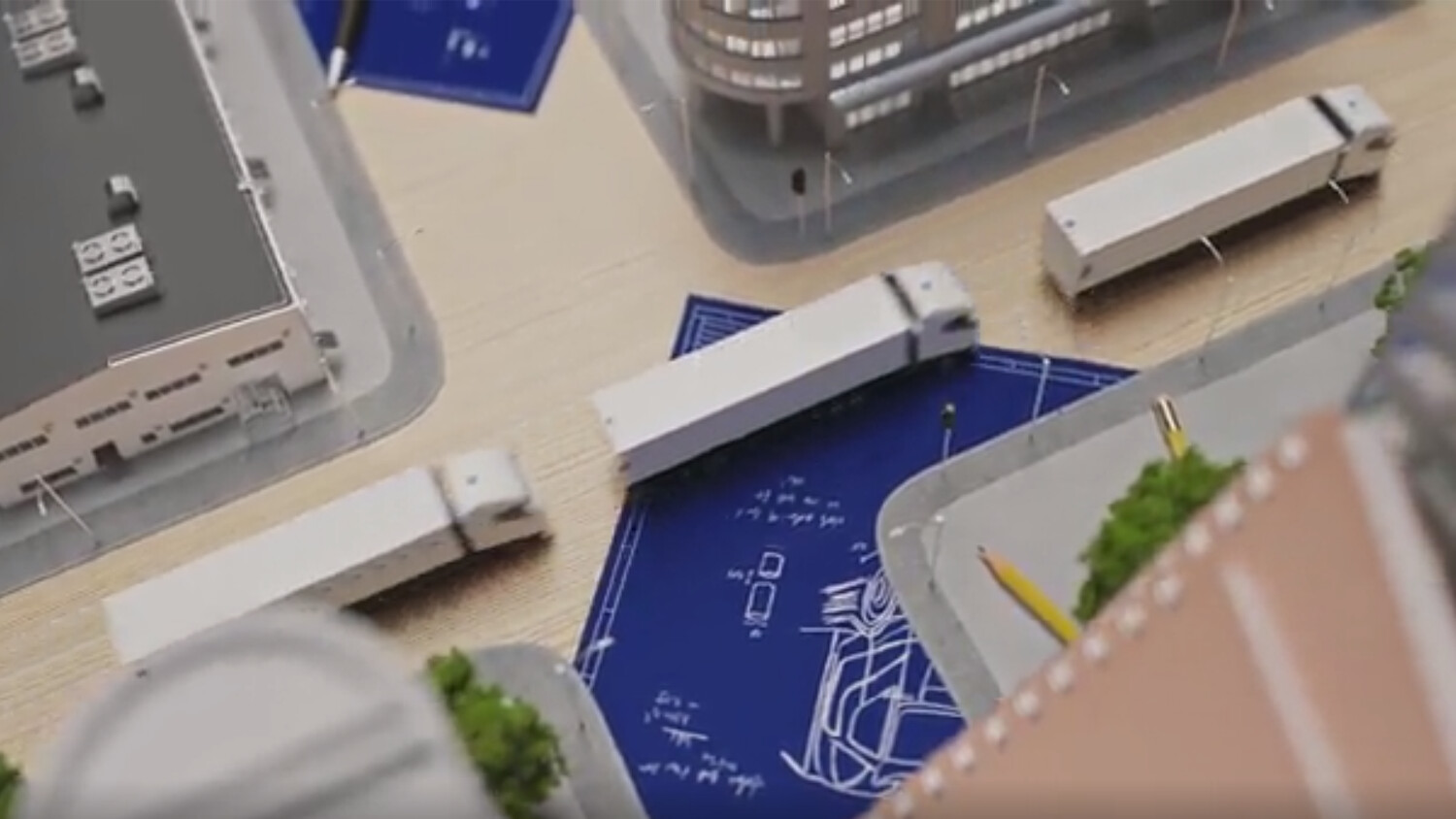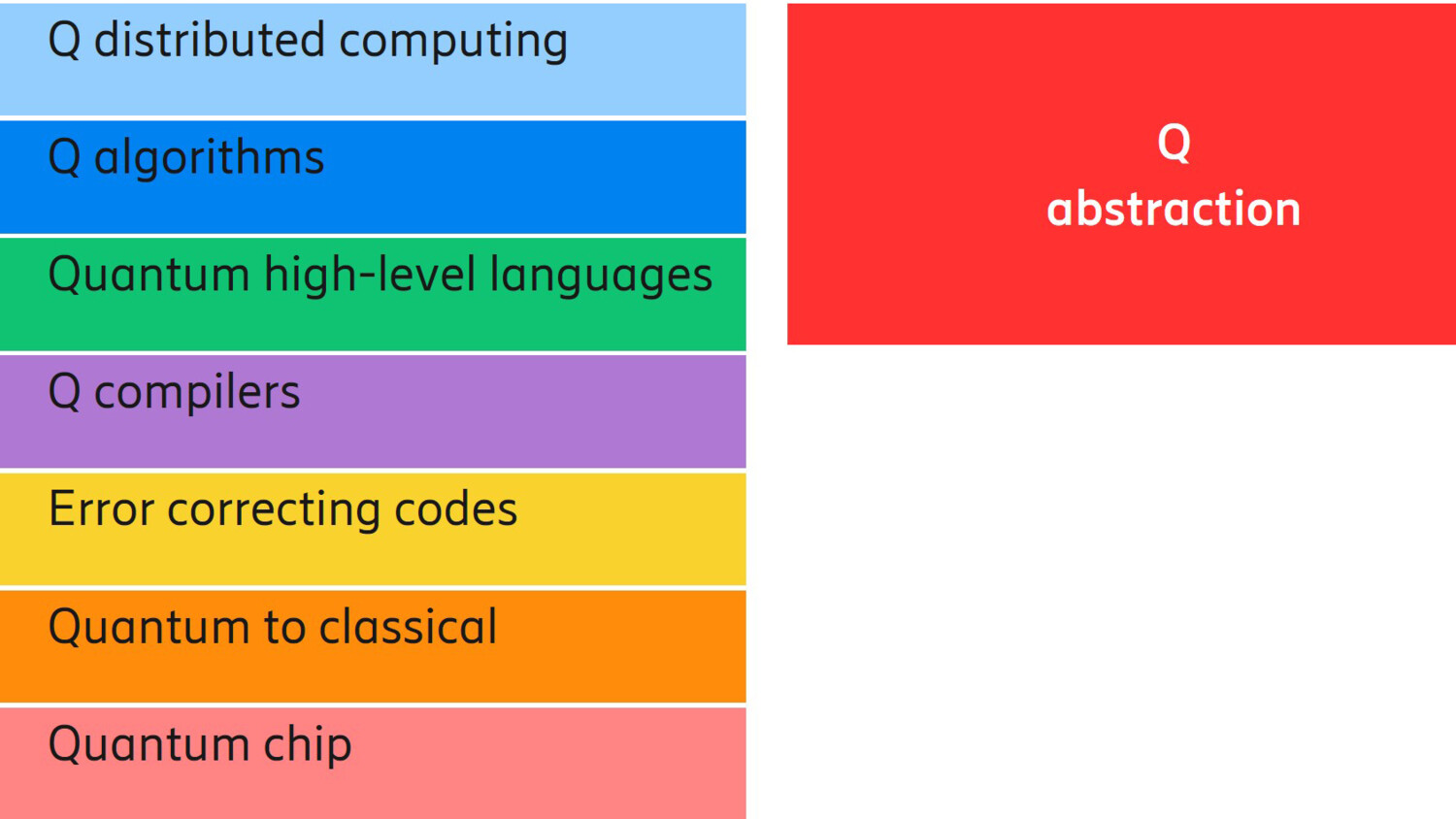# An introduction to quantum computer technology###### Jul 25, 2019 | 4 min.
Contributor (+2)
Category & Hashtags
###### Hashtags
#Quantumcomputing #EricssonResearch

In 5G, cloud technologies have become a critical part of radio access network (RAN) management e.g. our 5G vRAN solution. The ability to orchestrate both equipment and functions is enabling more automatized and intelligent forms of network management, from network set up to alarm management, license renewal and more. Such tasks, for example,  can now be performed more efficiently by intelligent agents that make optimised decisions in real time and under unpredictable circumstances.

However, those intelligent agents need to analyze sheer volume of data rates. The compute power required for such tasks surpass the capabilities of even the most state-of-art devices we have today. One solution comes in the form of quantum computer technology.

### Quantum computing vs. classical computing

Built using a quantum processor, a quantum computer (QC) can potentially reduce execution time to hours and days for problems that would previously have taken hundreds of years to solve on our best supercomputers, also known as classical computers. The basic element of a QC is the quantum bit (known as a qubit). Quantum bits are the quantum analog of the classical bits and thus the basic unit of quantum information. Whereas in the classical domain, they function as a two-level system, in this case, the qubits must obey the laws of quantum mechanics.

### The building blocks of quantum mechanics

The birth of quantum mechanics took place the first 27 years of the twentieth century to overcome the severe limitations in the validity of classical physics, with the first inconsistency being the Plank’s radiation law. Einstein, Debye, Bohr, de Broglie, Compton, Heisenberg, Schrödinger, Dirac amongst others were the pioneers in developing the theory of quantum mechanics as we know it today.

The fundamental building blocks of quantum mechanics are:

1. Quantisation: energy, momentum, angular momentum and other physical quantities of a bound system are restricted to discrete values (quantised)
2. Wave-particle duality: objects are both waves and particles
3. Heisenberg principle: the more precise the position of some particle is determined, the less precise its momentum can be known, and vice versa. Thus there is a fundamental limit to the measurement precision of physical quantities of a particle
4. Superposition: two quantum states can be added together, and the result is another valid quantum state
5. Entanglement: when the quantum state of any particle belonging to a system cannot be described independently of the state of the other particles, even when separated by a large distance, the particles are entangled
6. Fragility: by measuring a quantum system we destroy any previous information. From this, it follows the no-cloning theorem that states: it is impossible to create an identical copy of an arbitrary unknown quantum state

### Qubits and quantum computer technology

Since the first idea of a quantum computer was proposed by Benioff, Manin, Feynman and Deutsch back in the 1980s [benioff- Deutsch], many technological advances have taken place. We can now count on 10+ technologies that industry and academia are looking into with the goal of building a quantum computer. Some of them are targeting a 'universal' quantum computer, others a specialised quantum machine that will speed up specific problems.

This is made possible through qubits. Qubits can be realised with multiple technologies, for example, quantum dots are structures that can confine and manipulate a single electron to be acted as a qubit [loss, watson]. Another way of manipulating the spin of an electron is done via nitrogen-vacancy centers in diamond. Transmon qubits are one type of superconducting qubits that use Josephson junctions to create a single magnetic flux for use as a qubit [koch]. Ion-based qubits use ion traps to store qubits in individual ionised atoms suspended in a vacuum and controlled using lasers and electric and magnetic fields [blatt]. Qubits can also be achieved by controlling the polarisation of photons, or the number of photons. Unlike classical computing, qubits can be in 1, 0 or in a superposition of 1 or 0 quantum states until we measure them. Following this logic, a pair of qubits can be in any quantum superposition of four states and three qubits will be in any superposition of 8 states. By extrapolation, we can generalise that n qubits will be in a superposition of up to n different quantum states simultaneously. It is this simultaneity property that leads us to a potential quantum advantage. If we can use this massive parallelism, we can compute many operations at the same time, thus inferring a time advantage. Besides qubits, and in order to operate a quantum computer, we need the full quantum hardware and software layer stack (see figure 1).Figure 1. Quantum computer hardware and software stack

### Quantum algorithms vs. classical algorithms

Residing on the hardware level, the quantum gates will execute the mathematical operations dictated by the quantum algorithm in the Q abstraction layer. In between, we have the specific implementation layers for quantum languages, compilers and also error correcting codes. As classical computers are distinct from quantum computers, so are the algorithms. A quantum algorithm is different from a classical algorithm in the sense that it is not fully deterministic. A quantum algorithm is probabilistic, which means that the correct solution comes with a certain known probability. However, if we have a QC that is million times faster than a classical computer, we can always repeat the calculation multiple times and use voting to choose the one that comes up most often. Because of this probabilistic nature, quantum variants of classical algorithms will need to be developed to be executed in a QC.

Most basic algorithms such as Fourier Transform and linear equations are needed for the RAN user data plane functionality and algorithms such as clustering, support vector machines and kernel methods, and principal component analysis are needed for the RAN management plane functionality. However, in order to operate these algorithms in a QC with specific quantum gates, we need a quantum version of the classical algorithm. As of today, not all the classical algorithms are described in the quantum world and only a few of them have a quantum counterpart.

In a following post we explore the quantum counterparts of some of the most basic algorithms used in the data and management planes of the radio access networks. Visit our author pages to view this or other related blog posts.

### References

• Benioff, Paul, The computer as a physical system: A microscopic quantum mechan- ical Hamiltonian model of computers as represented by Turing machines, Journal of Statistical Physics. 22 (5) (1980)
• Manin, Yu. I. . Vychislimoe i nevychislimoe [Computable and Noncomputable] (in Russian). Sov.Radio. pp. 13-15. (1980)
• Feynman, R. P.u, Simulating physics with computers, International Journal of Theoretical Physics. 21 (6): 467-488 (1982)
• Deutsch, David, Quantum Theory, the Church-Turing Principle and the Universal Quantum Computer, Proceedings of the Royal Society of London A. 400(1818) (1985)
• D. Loss and D. P. DiVincenzo, Quantum computation with quantum dots, Phys. Rev. A, Gen. Phys., vol. 57, no. 1, pp. 120?126, 1998, doi: 10.1103/PhysRevA.57.120.
• T. F. Watson, S. G. J. Philips, E. Kawakami, D. R. Ward, P. Scarlino, M. Veldhorst, D. E. Savage, M. G. Lagally, M. Friesen, S. N. Coppersmith, M. A. Eriksson, and L. M. K. Vandersypen, A programmable two-qubit quantum processor in silicon, Nature, vol. 555, no. 7698, pp. 633?637, Mar. 2018, doi: 10.1038/nature25766
• J. Koch et al., ”Charge-insensitive qubit design derived from the Cooper pair box,” Phys. Rev. A 76, 04319 (2007)
• R. Blatt; D. J. Wineland ”Entangled states of trapped atomic ions” Nature. 453, 7198 (2008)